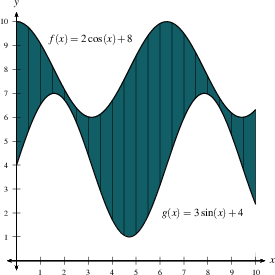Home

#### The Fundamental Theorem of Calculus

Three Different Concepts
The Fundamental Theorem of Calculus (Part 2)
The Fundamental Theorem of Calculus (Part 1)
More FTC 1

#### The Indefinite Integral and the Net Change

Indefinite Integrals and Anti-derivatives
A Table of Common Anti-derivatives
The Net Change Theorem
The NCT and Public Policy

#### Substitution

Substitution for Indefinite Integrals
Examples to Try
Revised Table of Integrals
Substitution for Definite Integrals
Examples

#### Area Between Curves

Computation Using Integration
To Compute a Bulk Quantity
The Area Between Two Curves
Horizontal Slicing
Summary

#### Volumes

Slicing and Dicing Solids
Solids of Revolution 1: Disks
Solids of Revolution 2: Washers
More Practice

#### Integration by Parts

Integration by Parts
Examples
Integration by Parts with a definite integral
Going in Circles
Tricks of the Trade

#### Integrals of Trig Functions

Antiderivatives of Basic Trigonometric Functions
Product of Sines and Cosines (mixed even and odd powers or only odd powers)
Product of Sines and Cosines (only even powers)
Product of Secants and Tangents
Other Cases

#### Trig Substitutions

How Trig Substitution Works
Summary of trig substitution options
Examples
Completing the Square

#### Partial Fractions

Introduction
Linear Factors
Improper Rational Functions and Long Division
Summary

#### Strategies of Integration

Substitution
Integration by Parts
Trig Integrals
Trig Substitutions
Partial Fractions

#### Improper Integrals

Type 1 - Improper Integrals with Infinite Intervals of Integration
Type 2 - Improper Integrals with Discontinuous Integrands
Comparison Tests for Convergence

#### Differential Equations

Introduction
Separable Equations
Mixing and Dilution

#### Models of Growth

Exponential Growth and Decay
Logistic Growth

#### Infinite Sequences

Approximate Versus Exact Answers
Examples of Infinite Sequences
Limit Laws for Sequences
Theorems for and Examples of Computing Limits of Sequences
Monotonic Covergence

#### Infinite Series

Introduction
Geometric Series
Limit Laws for Series
Test for Divergence and Other Theorems
Telescoping Sums and the FTC

#### Integral Test

The Integral Test
Estimates of Value of the Series

#### Comparison Tests

The Basic Comparison Test
The Limit Comparison Test

#### Convergence of Series with Negative Terms

Introduction, Alternating Series,and the AS Test
Absolute Convergence
Rearrangements

The Ratio Test
The Root Test
Examples

#### Strategies for testing Series

Strategy to Test Series and a Review of Tests
Examples, Part 1
Examples, Part 2

#### Power Series

Radius and Interval of Convergence
Finding the Interval of Convergence
Power Series Centered at $x=a$

#### Representing Functions as Power Series

Functions as Power Series
Derivatives and Integrals of Power Series
Applications and Examples

#### Taylor and Maclaurin Series

The Formula for Taylor Series
Taylor Series for Common Functions
Adding, Multiplying, and Dividing Power Series
Miscellaneous Useful Facts

#### Applications of Taylor Polynomials

Taylor Polynomials
When Functions Are Equal to Their Taylor Series
When a Function Does Not Equal Its Taylor Series
Other Uses of Taylor Polynomials

#### Partial Derivatives

Visualizing Functions in 3 Dimensions
Definitions and Examples
An Example from DNA
Geometry of Partial Derivatives
Higher Order Derivatives
Differentials and Taylor Expansions

#### Multiple Integrals

Background
What is a Double Integral?
Volumes as Double Integrals

#### Iterated Integrals over Rectangles

How To Compute Iterated Integrals
Examples of Iterated Integrals
Cavalieri's Principle
Fubini's Theorem
Summary and an Important Example

#### Double Integrals over General Regions

Type I and Type II regions
Examples 1-4
Examples 5-7
Order of Integration

### The Area Between Two Curves

To get the area between two curves, $f$ and $g$, we slice the region between them into vertical strips, each of width $\Delta x$.  Denote by $H(x)$ the height of the area at a point $x$.  The area of each strip is roughly $H(x)\cdot \Delta x$.
Adding up the area strips, the total area is approximately $\displaystyle\sum_{i=1}^n H(x_i)\, \Delta x$.  Taking a limit, the total area is exactly $\displaystyle\int_a^b H(x)\, dx$.

Let's look at an example.  Suppose $f(x)\ge g(x)$ for all $x$ in the interval we are considering, as in the graph below, so that $H(x)=f(x)-g(x)$ on the interval $[0,10]$.Then $$\displaystyle\int_a^b H(x)\, dx=\int_0^{10} (f(x)-g(x))\,dx=\int_0^{10}(2\cos x+8 -(3\sin x+4))\,dx=\int_0^{10}(2\cos x-3\sin x+4)\,dx.$$
DO:  Before looking ahead, evalute the previous integral.  Check your antiderivative!

$$\int_0^{10}(2\cos x-3\sin x+4)\,dx=(2\sin x+3\cos x+4x)\left|\begin{array}{c} ^{10} \\ _0 \end{array}\right .=2\sin(10)+3\cos(10)+4(10)-(2\cdot 0+3\cdot 1+4(0))$$$$=2\sin(10)+3\cos(10)+37\approx 2(-.544)+3(-.839)+37=33.395$$ (here we must round off the trig values, so our answer is no longer exact).

We look at more examples in the video below.  Notice while watching that, as was true in the example above, you must know what the graphs of the two functions look like in order to know which function is the larger.  Also notice that sometimes we are given the beginning and ending values of $x$ explicitly, and sometimes we have to figure out where two curves meet.